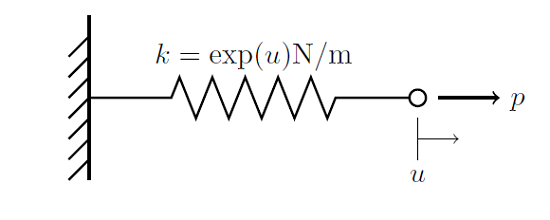# 通过递增非线性改进非线性问题的收敛

2013年 12月 3日

### 非线性递增示例f(u)=p-\exp(u)u

k(u,\beta)= k(u_0)+\beta \left[ k(u) – k(u_0) \right]

k(u,\beta)=\exp(u_0)+\beta\left[\exp(u)-\exp(u_0)\right]